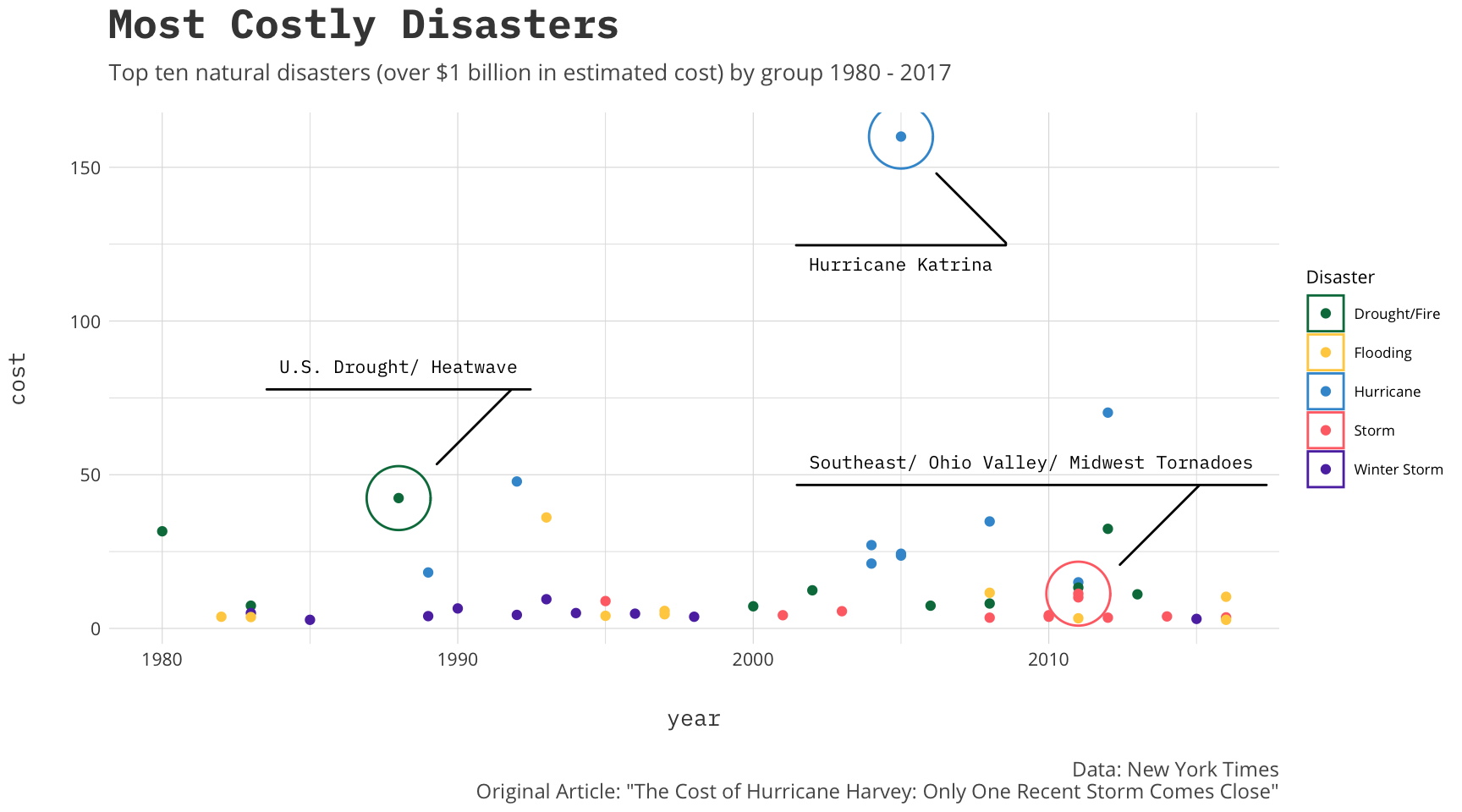On this website I use `awtools` which is a light (read not fully built) aesthetics package for all the charts and visuals. Every once in awhile I like to make tweaks so I thought I could take a minute to display some of the edits I made. Most the changes are to the color palettes, but there are a few spacing edits as well as tweaks to dark theme so why not makes a few charts. To do this we are going to need some data. Not too long ago I came across an article, The Cost of Hurricane Harvey: Only One Recent Storm Comes Close, which was discussed at length on Twitter for its visualization merit and I have long been interested in the data so that seems like a great dataset to revisit.

``````library(rvest)
library(ggplot2)
library(dplyr)
library(awtools)
library(ggbeeswarm)
library(ggforce)

#Natural Disasters from the NYT article
sep='\t',
stringsAsFactors = FALSE)

costs <- disasters %>%
mutate(
Disaster=case_when(
col2 == '#397dc2' ~ 'Hurricane',
col2 == '#efba2b' | col2 == '#9b0e11' ~ 'Drought/Fire',
col2 == '#699d8f' ~ 'Flooding',
col2 == '#9d76b0' ~ 'Storm',
col2 == '#61c6e2' ~ 'Winter Storm'
)
)``````

Now that the data is grouped I think the first question I would want to answer is a simple one: what does the distribution of disasters look like? I will group by disasters then plot them along a timeline so why not also use the new `a_dark_theme` which is my default theme using the IBM Plex Mono with a dark background and a couple other minor tweaks. We can size by the cost of the disaster and try to see if we notice any trends.

``````ggplot(costs, aes(x=year,y=Disaster,color=Disaster))+
ggbeeswarm::geom_quasirandom(alpha=.75,aes(size=cost),groupOnX = FALSE, show.legend = FALSE)+
a_dark_theme() +
a_main_color() +
labs(title='Billion Dollar Natural Disasters',
subtitle='The most costly naturdal disasters from 1980 - 2017',
y='',
caption='Data: New York Times\nOriginal Article: "The Cost of Hurricane Harvey: Only One Recent Storm Comes Close"')``````Although it looks like there may be a slight upward trend in number of disasters overall there are clear increases in the Drought/Fire and Storm groups. This makes me ask then what do these sorts of trends do to the cost of the disasters, maybe something like a running total of cost of disaster year over year.

We already have the data so let’s do it.

``````total.cost <- costs %>%
group_by(Disaster) %>%
arrange(Disaster,year) %>%
mutate(total = cumsum(cost))``````

Now that we have the running total of cost we need to display it. I think some sort of hybrid line chart that also has points indicating the disasters themselves.

``````#running total
ggplot(total.cost, aes(x=year,
y=total,
fill = Disaster,
group = Disaster)) +
geom_line(size=.5,aes(color=Disaster)) +
geom_point(aes(color=Disaster)) +
a_plex_theme() +
a_main_fill() +
a_main_color() +
labs(title='Natural Disasters and Runaway Cost',
subtitle='Running total of cost of natural disasters (over \$1 billion in estimated cost) from 1980 - 2017',
caption='Data: New York Times\nOriginal Article: "The Cost of Hurricane Harvey: Only One Recent Storm Comes Close"')``````I see that the cost of hurricanes (even if I were to remove Katrina) have been rising a bit more rapidly than the other groups. Similarly we see that within the groups the steps seem to get larger since 2010.

My final question I want to ask of the data is a bit self serving and was inspired by this tweet.

In this release there are tons of awesome new features but one I wanted to try was the new marking geoms that are included. To do this I will grab the top ten disasters in cost by group and plot them out over the years.

``````top.ten<-costs %>%
group_by(Disaster) %>%
top_n(n = 10, wt = cost)

labels<-costs %>%
group_by(Disaster) %>%
top_n(n = 1, wt = cost) %>%
filter(!Disaster %in% c('Winter Storm','Flooding'))``````

Now we can use the new `geom_mark_circle` to label a few.

``````#running total
ggplot(top.ten, aes(x=year, y=cost, color = Disaster, group = Disaster)) +
geom_point() +
geom_mark_circle(data=labels, aes(label=name,
color=Disaster,
fill=NA),
label.family = 'IBM Plex Mono',
label.fontsize = 8,
label.fill = NA,
label.fontface = 'plain') +
a_plex_theme() +
a_main_fill() +
a_main_color() +
labs(title='Most Costly Disasters',
subtitle='Top ten natural disasters (over \$1 billion in estimated cost) by group 1980 - 2017',
caption='Data: New York Times\nOriginal Article: "The Cost of Hurricane Harvey: Only One Recent Storm Comes Close"')``````